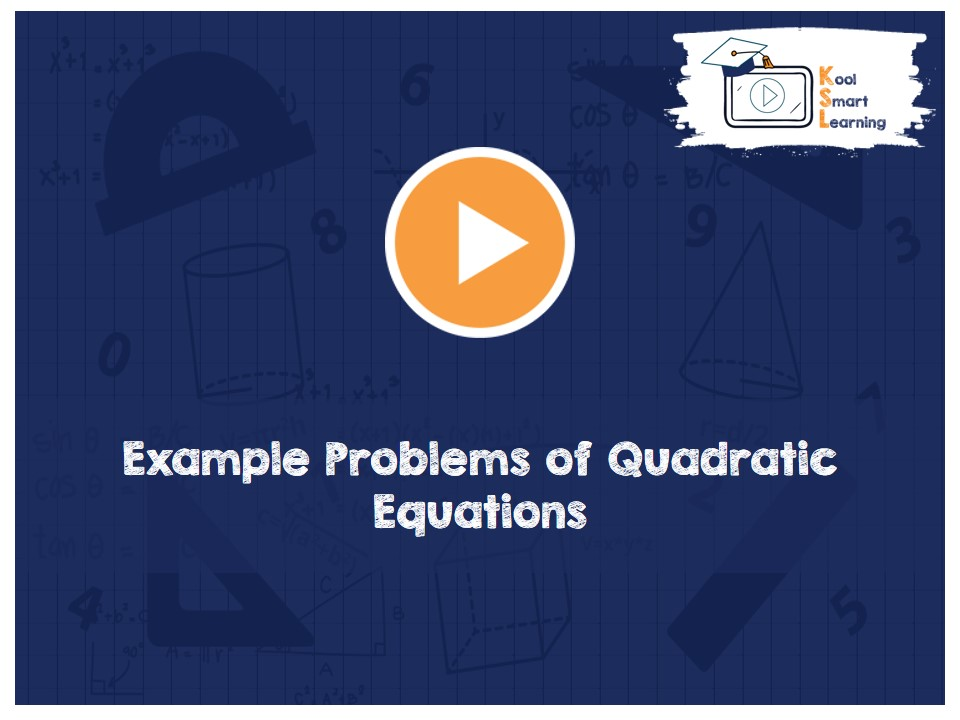At KoolSmartLearning, we intend to harness the power of online education to make learning easy.## Example Problems of Quadratic Equations

/  Example Problems of Quadratic Equations## Example Problems of Quadratic Equations

The video covers example problems of quadratic equations along with their solutions. The examples include questions on forming quadratic equation, solving an expression by changing it to quadratic equation, solving other equations (which don’t seem to be quadratic) by converting them to quadratic form besides solving the regular quadratic equation examples.

More Related Videos